Next: Endomorphism Graphs Up: Complexity Previous: Complexity

## Basic Issues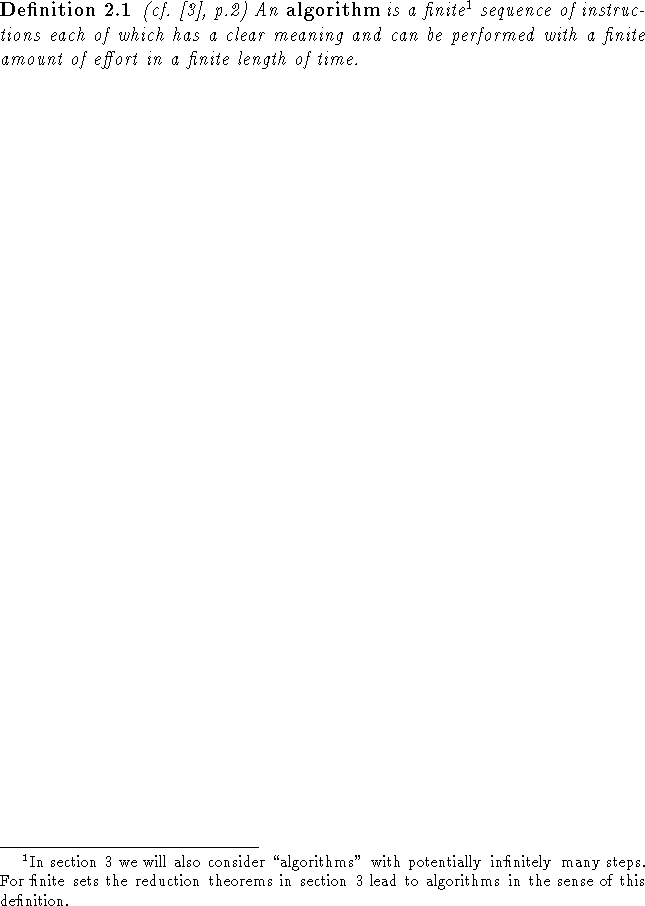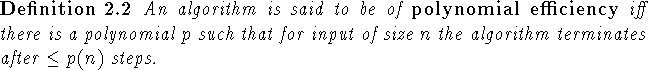Obviously answering the question ``Does P have the fixed point property?" for arbitrary P is a hard problem as one would need to check every order-preserving map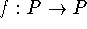for a fixed point. Since every ordered set P has at least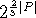order-preserving self maps (cf. ) this approach obviously is exponentially hard. (In fact computational data such as presented for example in  indicates that ordered sets should have many more order-preserving self-maps than this lower bound indicates.) Thus we formally have to reformulate the problem to: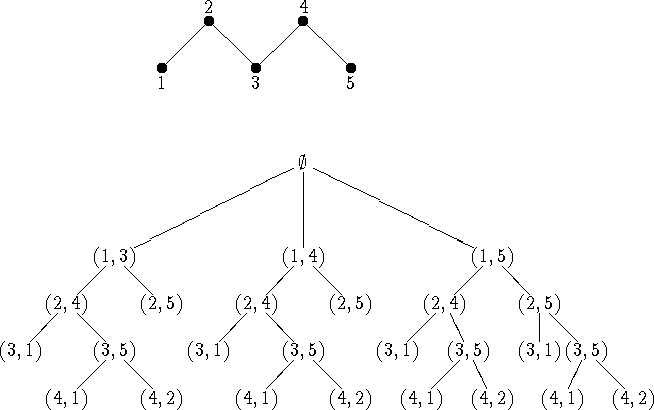Figure 1: The depth-first search tree in searching for a fixed point free order-preserving map of a five element fence.

This can be viewed as the NP (cf. Definition 2.12) version of the fixed point problem. Let us first consider the most simple-minded approach to the problem. (This approach for a reduced tree is described in the second half of . Similar programs were used on the whole tree to count order-preserving mappings in .) This will lead us to a very nice algorithm by Xia and some results that show the similarity between the problem of finding a fixed point free map and finding a fixed point free automorphism. For the actual proof of the NP-completeness of problem 2.3 the reader is referred to .
First one realizes that for a fixed point free order-preserving mapon a finite ordered set P we must have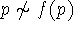for all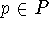. (Otherwise, say,implies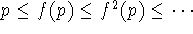and this iteration process must reach a fixed point in finitely many steps.) Now number the points of P from 1 to |P|. For every point k let the set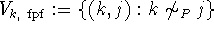be ordered by the natural order of the second component. Attempting to construct a fixed point free order-preserving map we can construct fixed point free order-preserving partial maps. We start withand add the first ordered pair in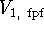to assign an image to 1. Then we add the first ordered pair in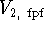to assign an image to 2. We continue until the partial map thus obtained is no longer order-preserving. Then we remove the last added ordered pair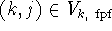and add instead the next ordered pair out of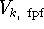. If there is no such pair, we remove the ordered pair (in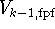) that was added second-to-last and continue as indicated (i.e., add the next pair inor remove the pair in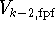that was last added). In this fashion we will find a fixed point free order-preserving map if there is one. The search tree for a simple example (a five element fence) is indicated in Figure 1. As one can see there is a lot of duplication in the tree. For example, in every branch it is the incompatibility of (3,1) with (2,4) which causes the first incident of ``backtracking". One might be able to save time if one could detect and use such incompatibilities earlier. This seems to be the idea of Xia's algorithm (cf. , resp. section 2.4, readers exclusively interested in Xia's algorithm can skip section 2.3).   We present these ideas in the following, choosing the language of graph theory rather than the language of formal concept analysis.Next: Endomorphism Graphs Up: Complexity Previous: Complexity

Bernd.S.W.Schroder